# Two gases X and Y are found in the atmosphere only trace amounts because they decompose quickly. When exposed to ultrav...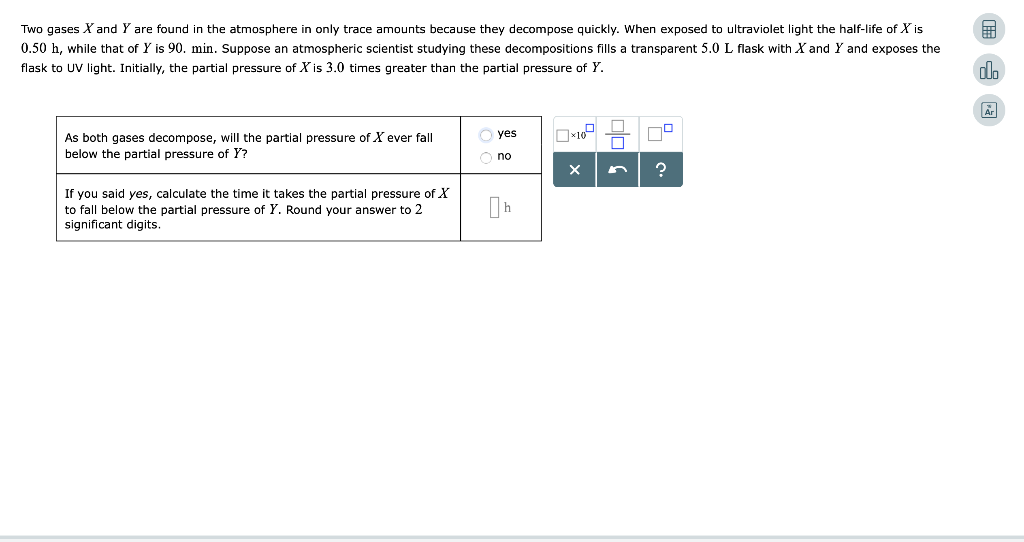Two gases X and Y are found in the atmosphere only trace amounts because they decompose quickly. When exposed to ultraviolet light the half-life of Xis 0.50 h, while that of Y is 90. min. Suppose an atmospheric scientist studying these decompositions fills a transparent 5.0 L flask with Xand Y and exposes the flask to UV light. Initially, the partial pressure of X is 3.0 times greater than the partial pressure of Y. dlo yes As both gases decompose, will the partial pressure of X ever fall below the partial pressure of Y? no X If you said yes, calculate the time it takes the partial pressure of X to fall below the partial pressure of Y. Round your answer to 2 significant digits.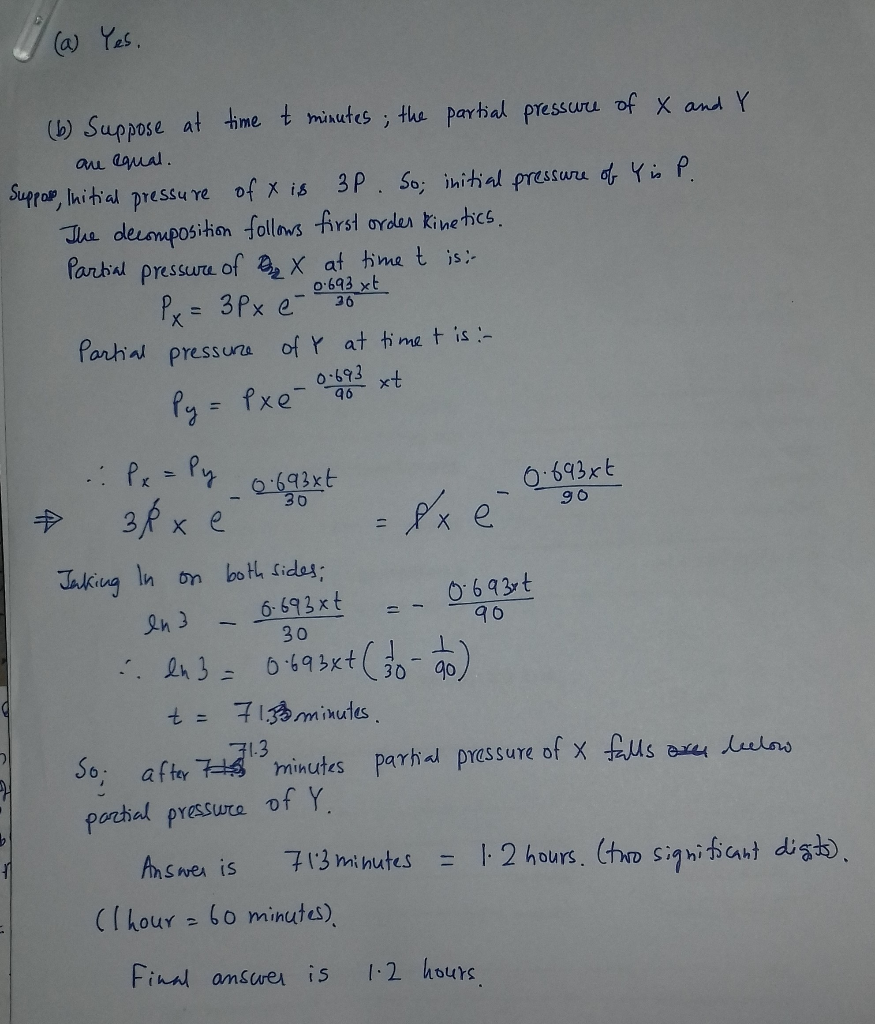##### Add Answer of: Two gases X and Y are found in the atmosphere only trace amounts because they decompose quickly. When exposed to ultrav...
Similar Homework Help Questions
• ### (20%) Problem 5: There are lots of examples of ideal gases in the universe, and they...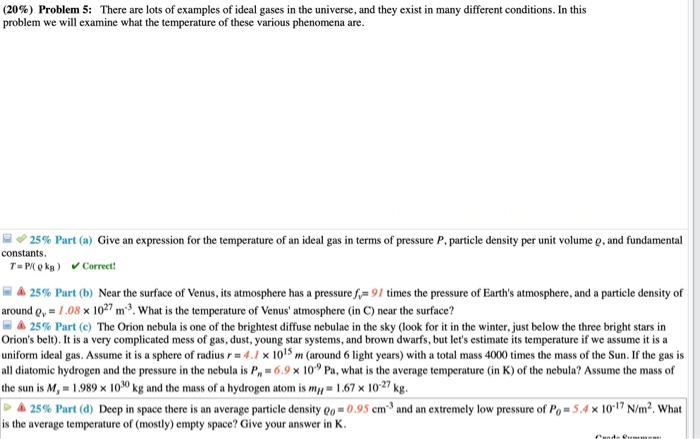(20%) Problem 5: There are lots of examples of ideal gases in the universe, and they exist in many different conditions. In this problem we will examine what the temperature of these various phenomena are. 25% Part (a) Give an expression for the temperature of an ideal gas in terms of pressure P. particle density per unit volume o, and fundamental constants. T=P k ) Correct! 25% Part (b) Near the surface of Venus, its atmosphere has a pressure f...

• ### (20% Problem 5: There are lots of examples of ideal gases in the universe, and they...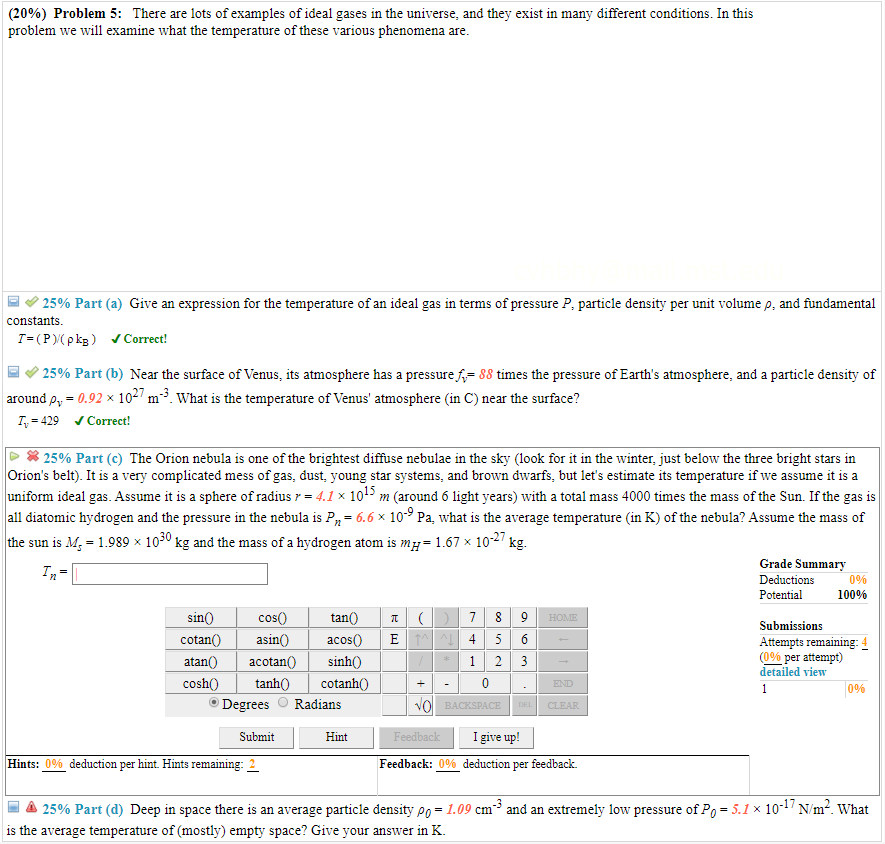(20% Problem 5: There are lots of examples of ideal gases in the universe, and they exist in many different conditions. In this problem we will examine what the temperature of these various phenomena are 25% Part (a) Give an expression for the temperature of an ideal gas in terms of pressure P, particle density per unit volume p, and fundamental constants T (P)(pkg vCorrect! 25% Part (b) Near the surface of Venus, its atmosphere has a pressure f 88...

• ### Equal masses of CO2 and CH4 gas are placed in identical 1.00 L containers, both at the same temperature. Which of the following is true?   X.   Both gases exert the same pressure.   Y.   Molecules of...

Equal masses of CO2 and CH4 gas are placed in identical 1.00 L containers, both at the same temperature. Which of the following is true?   X.   Both gases exert the same pressure.   Y.   Molecules of CH4 make more frequent collisions with the walls of the container.   Z.   Both gases have the same average molecular speed. answer can be more than one

• ### biomaterials 2. You are performing an in vitro test in which you are testing the adsorption of three proteins (X, Y, and Z) to a material. After you have exposed the proteins to...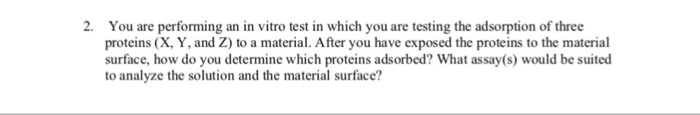biomaterials 2. You are performing an in vitro test in which you are testing the adsorption of three proteins (X, Y, and Z) to a material. After you have exposed the proteins to the material surface, how do you determine which proteins adsorbed? What assay(s) would be suited to analyze the solution and the material surface 2. You are performing an in vitro test in which you are testing the adsorption of three proteins (X, Y, and Z) to a...

• ### Define a relation R from Z to Z by (x,y) are in the relation if and only if the absolute value of (x-y) is less than or equal to two

Define a relation R from Z to Z by (x,y) are in the relation if and only if the absolute value of (x-y) is less than or equal to two. Is R symmetric?

Need Online Homework Help?